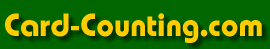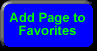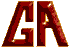## Blackjack Outcome Calculator

Here, you can estimate the probability of winning or losing a specified amount of money given betsize, number of hands and advantage percentage. To find your advantage at Basic Strategy, link to the Blackjack Advantage Calculator. To use this calculator, fill in the four boxes below and press the Calculate button. Your expected outcome, standard deviation above or below the desired outcome and probability that you will win the desired amount will be displayed. Placing a zero in the desired outcome box will give you your probability of winning.

Enter the player advantage percentage (typically -1 to +0.5)

Enter the number of hands you will play

Enter the average bet size in dollars

Enter the desired outcome in dollars

 Expected outcome in units Expected outcome in dollars The standard deviation from desired outcome Probability percentage of winning at least the desired amount

Toll Free Number
800-498-2234
8am-6pm ESTgamblersanonymous.org